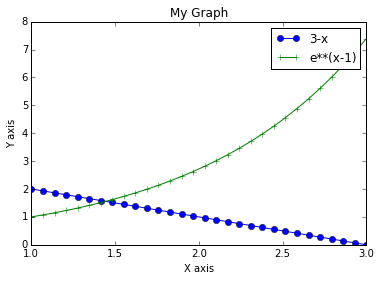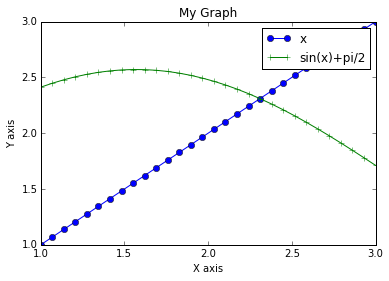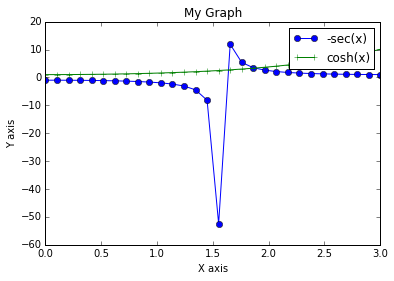# Chapter 1: Solution of Equation & Curve Fitting¶

## Example 1.1, page no. 21¶

In :
import numpy

x = numpy.poly()
p = 2*(x**3)+x**2-13*x+6
print "The roots of above equation are: ", numpy.roots([2,1,-13,6])

The roots of above equation are:  [-3.   2.   0.5]


## Example 1.2, page no. 21¶

In :
import numpy

x = numpy.poly()
print "The roots of the equation are: ", numpy.roots ([3, -4, 1, 88])

The roots of the equation are:  [ 2.00000000+2.64575131j  2.00000000-2.64575131j -2.66666667+0.j        ]


## Example 1.3, page no. 22¶

In :
import numpy

x = numpy.poly()
p = x^3-7*(x^2)+36
print "The roots of above equation are:", numpy.roots([1, -7, 0, 36])

The roots of above equation are: [ 6.  3. -2.]


## Example 1.4, page no. 23¶

In :
import numpy

x = numpy.poly ()
p = x**4-2*(x**3)-21*(x**2)+22*x+40
print "The roots of above equation are:", numpy.roots([1,-2,-21,22,40])

The roots of above equation are: [ 5. -4.  2. -1.]


## Example 1.5, page no. 23¶

In :
import numpy

x = numpy.poly ()
p = 2*(x**4)-15*(x**3)+35*(x**2)-30*x+8
print "The roots of above equation are:", numpy.roots([2,-15,35,-30,8])

The roots of above equation are: [ 4.   2.   1.   0.5]


## Example 1.6, page no. 24¶

In :
import numpy
import sympy

x = numpy.poly ()
p = x**3-3*(x**2)+1
ans = numpy.roots([1,-3, 0, 1])
print "The roots of above equation are:", ans
x = sympy.Symbol('x')
print "let x1 = 0.6527036 x2 = -0.5320889 x3 = 2.8793852"
print "So the equation whose roots are cube of the roots of above equation is (x−x1ˆ3)∗(x−x2ˆ3)∗(x−x3ˆ3)"
p1 = (x-x1**3)*(x-x2**3)*(x-x3**3)
print p1

The roots of above equation are: [ 2.87938524  0.65270364 -0.53208889]
let x1 = 0.6527036 x2 = -0.5320889 x3 = 2.8793852
So the equation whose roots are cube of the roots of above equation is (x−x1ˆ3)∗(x−x2ˆ3)∗(x−x3ˆ3)
(x - 23.8725770741465)*(x - 0.278066086195109)*(x + 0.150644263115026)


## Example 1.7, page no. 25¶

In :
import numpy
import sympy

x = numpy.poly ()
x1 = numpy.poly ()
x2 = numpy.poly ()
x3 = numpy.poly ()
x4 = numpy.poly ()
x5 = numpy.poly ( )
x6 = numpy.poly ()
p = x**3-6*(x**2)+5*x+8
print "The roots of above equation are:"
print numpy.roots ([1, -6, 5, 8])
print "let x1 = -0.7784571 x2 = 2.2891685 x3 = 4.4892886"
x1 = -0.7784571
x2 = 2.2891685
x3 = 4.4892886
print "Now, since we want equation whose sum of roots is 0. sum of roots of above equation is 6, so we will decrease"
print "Value of each root by 2 i.e. x4 = x1-2"
x = sympy.Symbol('x')
x4 = x1-2
print "x4 = ", x4
x5=x2-2
print "x5 = ", x5
x6=x3-2
print "x6 = ", x6
print "Hence, the required equation is ( x−x4 ) ∗ ( x−x5 ) ∗ ( x−x6 ) = 0 −−>"
p1 =( x-x4 )*(x-x5)*(x-x6)
print p1

The roots of above equation are:
[ 4.48928857  2.28916855 -0.77845712]
let x1 = -0.7784571 x2 = 2.2891685 x3 = 4.4892886
Now, since we want equation whose sum of roots is 0. sum of roots of above equation is 6, so we will decrease
Value of each root by 2 i.e. x4 = x1-2
x4 =  -2.7784571
x5 =  0.2891685
x6 =  2.4892886
Hence, the required equation is ( x−x4 ) ∗ ( x−x5 ) ∗ ( x−x6 ) = 0 −−>
(x - 2.4892886)*(x - 0.2891685)*(x + 2.7784571)


## Example 1.8, page no. 28¶

In :
import numpy

x = numpy.poly ()
p = 6*(x**5)-41*(x**4)+97*(x**3)-97*(x**2)+41*x-6
print "The roots of above equation are:",numpy.roots([6,-41,97,-97,41,-6])

The roots of above equation are: [ 3.          2.          1.          0.5         0.33333333]


## Example 1.9, page no. 28¶

In :
import numpy

x = numpy.poly()
p = 6*(x**6)-25*(x**5)+31*(x**4)-31*(x**2)+25*x-6
print "The roots of above equation are:", numpy.roots([6,-25,31,0,-31,25,-6])

The roots of above equation are: [ 2.00000000+0.j        -1.00000000+0.j         0.83333333+0.5527708j
0.83333333-0.5527708j  1.00000000+0.j         0.50000000+0.j       ]


## Example 1.10, page no. 29¶

In :
import numpy

x = numpy.poly ()
p = x**3-3*(x**2)+12*x+16
print "The roots of above equation are:", numpy.roots([1,-3,12,16])

The roots of above equation are: [ 2.+3.46410162j  2.-3.46410162j -1.+0.j        ]


## Example 1.11, page no. 30¶

In :
import numpy
x = numpy.poly ()
p = 28*(x**3)-9*(x**2)+1
print "The roots of above equation are:",numpy.roots([28,-9,0,1])

The roots of above equation are: [ 0.28571429+0.24743583j  0.28571429-0.24743583j -0.25000000+0.j        ]


## Example 1.12, page no. 31¶

In :
import numpy

x = numpy.poly ()
p = x**3+x**2-16*x+20
print "The roots of above equation are:",numpy.roots ([1,1,-16,20])

The roots of above equation are: [-5. +0.00000000e+00j  2. +2.90013456e-08j  2. -2.90013456e-08j]


## Example 1.13, page no. 31¶

In :
import numpy

x = numpy.poly ()
p = x**3-3*(x**2)+3
print "The roots of above equation are:",numpy.roots ([1,-1,0,3])

The roots of above equation are: [ 1.08727971+1.17131211j  1.08727971-1.17131211j -1.17455941+0.j        ]


## Example 1.14, page no. 33¶

In :
import numpy

x = numpy.poly()
p = x**4-12*(x**3)+41*(x**2)-18*x-72
print "The roots of above equation are:",numpy.roots ([1,-12,41,-18,-72])

The roots of above equation are: [ 6.  4.  3. -1.]


## Example 1.15, page no. 34¶

In :
import numpy

x = numpy.poly ()
p = x**4-2*(x**3)-5*(x**2)+10*x-3
print "The roots of above equation are:", numpy.roots ([1,-2,-5,10,-3])

The roots of above equation are: [-2.30277564  2.61803399  1.30277564  0.38196601]


## Example 1.16, page no. 35¶

In :
import numpy

x = numpy.poly ()
p = x**4-8*(x**2)-24*x+7
print "The roots of above equation are:",numpy.roots ([1,0,-8,-24,7])

The roots of above equation are: [ 3.73205081+0.j         -2.00000000+1.73205081j -2.00000000-1.73205081j
0.26794919+0.j        ]


## Example 1.17, page no. 35¶

In :
import numpy

x = numpy.poly ()
p = x**4-6*(x**3)-3*(x**2)+22*x-6
print "The roots of above equation are:",numpy.roots ([1,-6,-3,22.-6])

The roots of above equation are: [ 6.05932014 -1.65491082  1.59559067]


## Example 1.18, page no. 37¶

In :
%matplotlib inline
import matplotlib.pyplot as plt
import numpy
import math

x = numpy.linspace(1 ,3 ,30)
y1 = 3-x
y2 = math.e**(x-1)
plt.xlabel('X axis')
plt.ylabel('Y axis')
plt.title('My Graph')
plt.plot(x, y1, "o-" )
plt.plot(x, y2, "+-" )
plt.legend(["3-x" ,"e**(x-1)"])
print "From the graph, it is clear that the point of intersection is nearly x = 1.43"

From the graph, it is clear that the point of intersection is nearly x = 1.43## Example 1.19, page no. 40¶

In :
%matplotlib inline
import matplotlib.pyplot as plt
import numpy
import math

x = numpy.linspace(1 ,3 ,30)
y1 = x
y2 = numpy.sin(x)+math.pi/2
plt.xlabel('X axis')
plt.ylabel('Y axis')
plt.title('My Graph')
plt.plot(x, y1, "o-")
plt.plot(x, y2, "+-")
plt.legend(["x", "sin(x)+pi/2"])
print "From the graph, it is clear that the point of intersection is nearly x=2.3"

From the graph, it is clear that the point of intersection is nearly x=2.3## Example 1.20, page no. 41¶

In :
%matplotlib inline
import matplotlib.pyplot as plt
import numpy
import math

x = numpy.linspace(0, 3, 30)
y1 = -1/numpy.cos(x)
y2 = numpy.cosh(x)
plt.xlabel('X axis')
plt.ylabel('Y axis')
plt.title('My Graph')
plt.plot(x, y1, "o-" )
plt.plot(x, y2, "+-" )
plt.legend (["-sec(x)", "cosh(x)"])
print "From the graph, it is clear that the point of intersection is nearly x=2.3 "

From the graph, it is clear that the point of intersection is nearly x=2.3